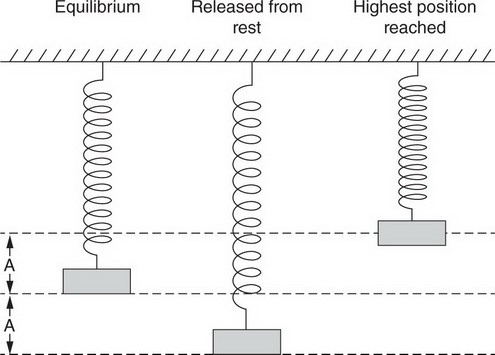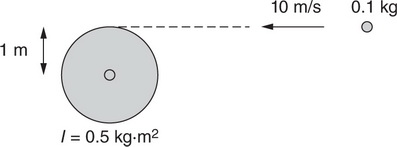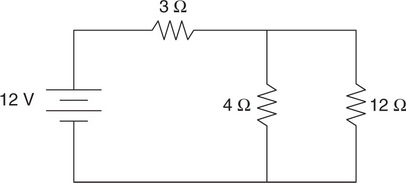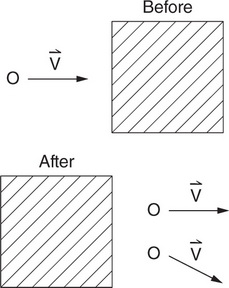# AP Physics 1 Practice Test 12

### Test Information10 questions18 minutes

1.A block is attached to a vertical spring. The block is pulled down a distance A from equilibrium, as shown above, and released from rest. The block moves upward; the highest position above equilibrium reached by the mass is less than A, as shown. When the mass returns downward, how far below the equilibrium position will it reach?

2.Two charged Styrofoam balls are brought a distance d from each other, as shown. The force on Ball B is 2 μN to the right. When the distance between the balls is changed, the force on Ball B is 8 μN to the right.

Which of the following can indicate the sign of the charges of balls A and B?

Ball A    Ball B

3.Two charged Styrofoam balls are brought a distance d from each other, as shown. The force on Ball B is 2 μN to the right. When the distance between the balls is changed, the force on Ball B is 8 μN to the right.

When the force on Ball B is 8 μN, what is the distance between the centers of the two balls?

4.A disk of radius 1 m and rotational inertia I = 0.5 kg.m2 is free to rotate, but initially at rest. A blob of putty with mass 0.1 kg is traveling toward the disk with a speed of 10 m/s, as shown in the preceding figure. The putty collides with the outermost portion of the disk and sticks to the disk. What is the angular momentum of the combined disk-putty system after the collision?

5. A 1-kg object is released from rest at the top of a rough-surfaced incline. The object slides without rotating to the bottom of the incline. The object’s kinetic energy at the bottom must be

6.What is the current in the 4 Ω resistor in the circuit in the preceding illustration?

7.Two sounds are played in the laboratory. A microphone is connected to an oscilloscope, which displays the traces shown above for each sound. On these traces, the horizontal axis is time; the vertical axis is related to the distance the microphone’s diaphragm is displaced from its resting position. The scales are identical for each diagram. Which of the following is correct about the sounds that produce the traces above?

8. The radius of Mars is about half that of Earth; the mass of Mars is about one-tenth that of Earth. Which of the following is closest to the gravitational field at the surface of Mars?

9.In an experiment, a marble rolls to the right at speed v, as shown in the top diagram. The marble rolls under a canopy, where it is heard to collide with marbles that were not initially moving. Such a collision is known to be elastic. After the collision, two equal-mass marbles are observed leaving the canopy with velocity vectors directed as shown. Which of the following statements justifies why the experimenter believes that a third marble was involved in the collision under the canopy?

10.In the laboratory, two carts on a track collide in the arrangement shown in the preceding figure. Before the collision, the 2-kg cart travels through photogate 1, which measures its speed; the 0.25-kg cart is initially at rest. After the collision, the carts bounce off one another. Photogate 2 measures the speed of each cart as it passes.

A student is concerned about his experimental results. When he adds the momentum of both carts after collision, he gets a value greater than the momentum of the 2-kg cart before collision. Which of the following is a reasonable explanation for the discrepancy?